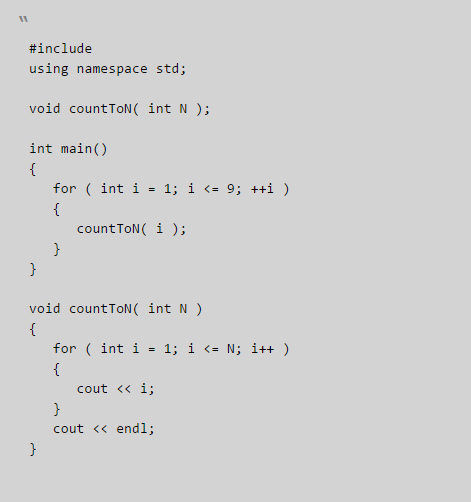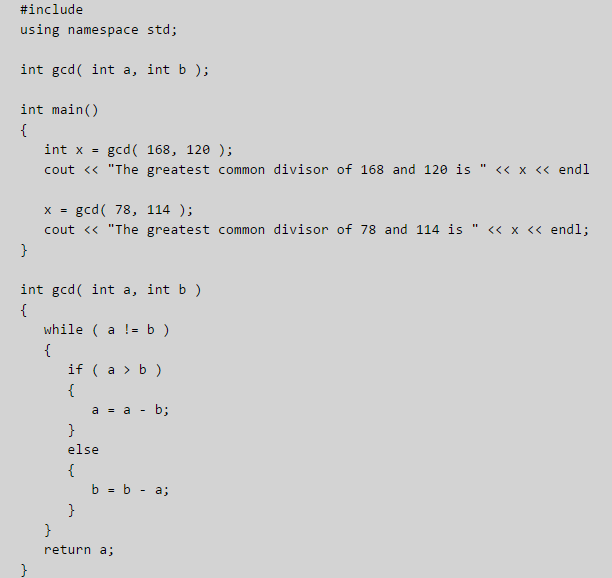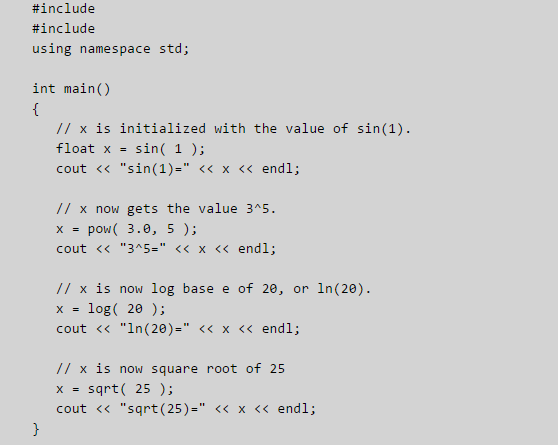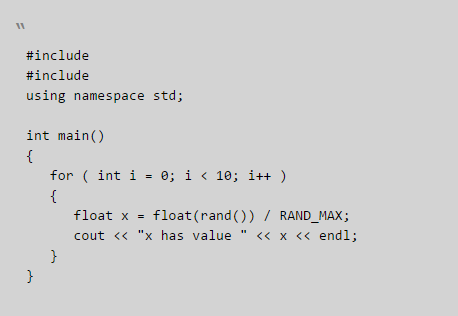# Answered! C++ program was resulting the value of 2 instead of 2.5 , what is the mistake ?…

C++ program was resulting the value of 2 instead of 2.5 , what is the mistake ?

void main() {

Don't use plagiarized sources. Get Your Custom Essay on
Answered! C++ program was resulting the value of 2 instead of 2.5 , what is the mistake ?…
GET AN ESSAY WRITTEN FOR YOU FROM AS LOW AS \$13/PAGE

intx = 5 ;

cout <<x/2 ;

}

The variable x is declared to be of int type. So the division results in an int result. For a result to be fraction, atleast on of the 2 operands have to be float / double.

So you can change your code as

cout << x / 2.0;

Here 2.0 is a double , where as x is int. Since atleast one of the operands is double, hence the output of division will also be double.

The other way of getting the fractional part is to make x of type double;

void main()

{

double x = 5;

cout<< x / 2;

}

In this case, since x is a double, the output of division will be double.

Hope the answer helps. Please do not forget to rate the answer if it helped. Thank you very much.